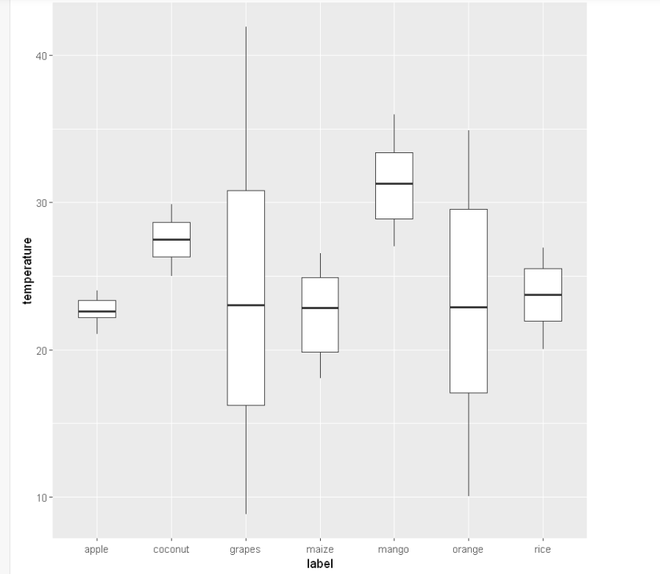Open in App
Not now

# Spacing between boxplots in ggplot2 in R

• Last Updated : 18 Jul, 2021

In this article, we are going to see how to add space between the boxplots in ggplot2 using R programming language.

Dataset in use: Crop_recommendation

### Method 1: Using width between boxplot

Here we will use width attributes to define space between the boxplot. In this the value is passed to the attribute.

Syntax: geom_boxplot(width)

Program:

## R

 `library``(ggplot2)`` ` `# loading data set and storing it in ds variable``df <- ``read.csv``(``"Crop_recommendation.csv"``, header = ``TRUE``)`` ` `# create a boxplot by using geom_boxplot() function``# of ggplot2 package``plot = ``ggplot``(data=df,``              ``mapping=``aes``(``                ``x=label, y=temperature))+``geom_boxplot``(width = 0.5)``plot `

Output:### Method 2: Using position_dodge

Here we will use position_dodge to define the vertical position of a geom while adjusting the horizontal position. position_dodge() requires the grouping variable to be specified position.

Syntax:

geom_boxplot( position = position_dodge(width))

Program:

## Python3

 `library(ggplot2)`` ` `# loading data set and storing it in ds variable``df <``-` `read.csv(``"Crop_recommendation.csv"``, header ``=` `TRUE)`` ` `# create a boxplot by using geom_boxplot() function``# of ggplot2 package``plot``=` `ggplot(data``=``df,``             ``mapping``=``aes(x``=``label, ``                         ``y``=``temperature))``+``geom_boxplot(width``=``0.1``, position ``=` `position_dodge(width``=``0.5``))``plot`

Output:My Personal Notes arrow_drop_up